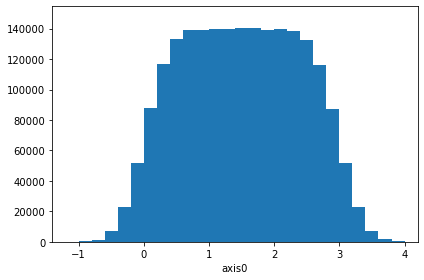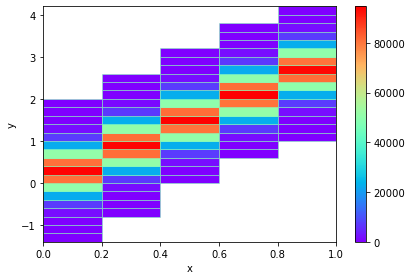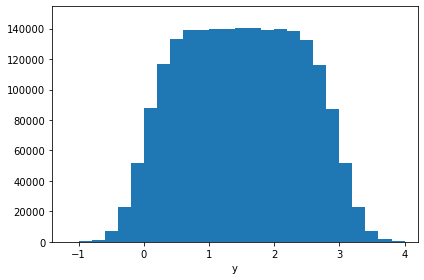# Support for dask arrays¶

It is possible to operate on dask arrays and spare the memory (or perhaps even time).

In :
# Necessary imports
import physt
import numpy as np

import dask.array as da
from physt import h1, h2
%matplotlib inline

In :
# Create two arrays
np.random.seed(42)

SIZE = 2 ** 21
CHUNK = int(SIZE / 16)

million = np.random.rand(SIZE)#.astype(int)
million2 = (3 * million + np.random.normal(0., 0.3, SIZE))#.astype(int)

# Chunk them for dask
chunked = da.from_array(million, chunks=(CHUNK))
chunked2 = da.from_array(million2, chunks=(CHUNK))


## Create histograms¶

h1, h2, ... have their alternatives in physt.dask_compat. They should work similarly. Although, they are not complete and unexpected errors may occur.

In :
from physt.compat.dask import h1 as d1
from physt.compat.dask import h2 as d2

In :
# Use chunks to create a 1D histogram
ha = d1(chunked2, "fixed_width", bin_width=0.2)
check_ha = h1(million2, "fixed_width", bin_width=0.2)
ok = (ha == check_ha)
print("Check: ", ok)
ha.plot()
ha

Check:  True

Out:
Histogram1D(bins=(28,), total=2097152, dtype=int64)In :
# Use chunks to create a 2D histogram
hb = d2(chunked, chunked2, "fixed_width", bin_width=.2, axis_names=["x", "y"])
check_hb = h2(million, million2, "fixed_width", bin_width=.2, axis_names=["x", "y"])
hb.plot(show_zero=False, cmap="rainbow")
ok = (hb == check_hb)
print("Check: ", ok)
hb

/home/honza/code/my/physt/physt/util.py:74: FutureWarning:

histogramdd is deprecated, use h instead


Check:  True

Out:
Histogram2D(bins=(5, 28), total=2097152, dtype=int64)In :
# And another cross-check
hh = hb.projection("y")
hh.plot()
print("Check: ", np.array_equal(hh.frequencies, ha.frequencies))   # Just frequencies

Check:  TrueIn :
# Use dask for normal arrays (will automatically split array to chunks)
d1(million2, "fixed_width", bin_width=0.2) == ha

Out:
True

## Some timings¶

Your results may vary substantially. These numbers are just for illustration, on 4-core (8-thread) machine. The real gain comes when we have data that don't fit into memory.

### Efficiency¶

In :
# Standard
%time h1(million2, "fixed_width", bin_width=0.2)

CPU times: user 412 ms, sys: 14.2 ms, total: 427 ms
Wall time: 426 ms

Out:
Histogram1D(bins=(28,), total=2097152, dtype=int64)
In :
# Same array, but using dask
%time d1(million2, "fixed_width", bin_width=0.2)

CPU times: user 751 ms, sys: 24.3 ms, total: 775 ms
Wall time: 199 ms

Out:
Histogram1D(bins=(28,), total=2097152, dtype=int64)
In :
# Most efficient: dask with already chunked data
%time d1(chunked2, "fixed_width", bin_width=0.2)

CPU times: user 685 ms, sys: 13.5 ms, total: 699 ms
Wall time: 153 ms

Out:
Histogram1D(bins=(28,), total=2097152, dtype=int64)

### Different scheduling¶

In :
%time d1(chunked2, "fixed_width", bin_width=0.2)

CPU times: user 573 ms, sys: 37.6 ms, total: 611 ms
Wall time: 135 ms

Out:
Histogram1D(bins=(28,), total=2097152, dtype=int64)
In :
%%time
# Hyper-threading or not?
graph, name = d1(chunked2, "fixed_width", bin_width=0.2, compute=False)

CPU times: user 442 ms, sys: 11.1 ms, total: 453 ms
Wall time: 140 ms

Out:
Histogram1D(bins=(28,), total=2097152, dtype=int64)
In :
# Multiprocessing not so efficient for small arrays?

CPU times: user 42.6 ms, sys: 158 ms, total: 201 ms

Histogram1D(bins=(28,), total=2097152, dtype=int64)